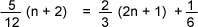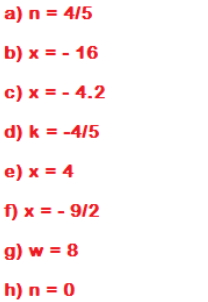Solve Linear Equations with Variables on Both Sides of the Equation

Solve Linear Equations with Variables

on Both Sides of the Equation

If an equation contains a variable on both sides of the equality sign, it will need to be rewritten so that the variable is only on one side of the equation.

Steps for Solving Linear Equations

1.  If the equation contains fractions, you may elect to multiply both sides of the equation by the least common denominator (LCD). That will eliminate fractions from the equation.

2.  Use the distributive property to remove parentheses.

3.  Combine like terms on the same side of the equality sign.

4.  Use the addition property to get the variable on one side of the equals sign and all terms that do not contain the variable on the other side.

5.  Use the multiplication property to isolate the variable.

6.  Check the solution by plugging your result into the original equation.

Example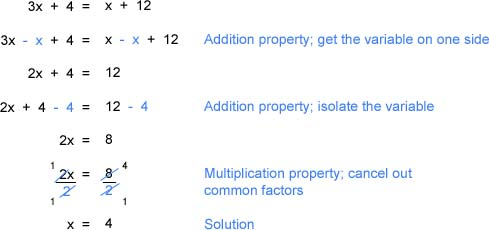Practice

Solve each equation.  Check your solutions.

a)  2(n + 3) = -3n + 10

b)  2(x - 5) + 3 = 3x + 9

c)  5.74x + 5.42 = 2.24x - 9.28

d)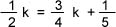e)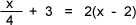f)  1/2 (2x + 3) = 2/3 (x - 6) + 4

g)  12 - 6w + 3(2w + 3) = 2w + 5

h)